February 18, 2017

Distributive Laws

Posted by Emily RiehlGuest post by Liang Ze Wong

The Kan Extension Seminar II continues and this week, we discuss Jon Beck’s “Distributive Laws”, which was published in 1969 in the proceedings of the Seminar on Triples and Categorical Homology Theory, LNM vol 80. In the previous Kan seminar post, Evangelia described the relationship between Lawvere theories and finitary monads, along with two ways of combining them (the sum and tensor) that are very natural for Lawvere theories but less so for monads. Distributive laws give us a way of composing monads to get another monad, and are more natural from the monad point of view.

Beck’s paper starts by defining and characterizing distributive laws. He then describes the category of algebras of the composite monad. Just as monads can be factored into adjunctions, he next shows how distributive laws between monads can be “factored” into a “distributive square” of adjunctions. Finally, he ends off with a series of examples.

Before we dive into the paper, I would like to thank Emily Riehl, Alexander Campbell and Brendan Fong for allowing me to be a part of this seminar, and the other participants for their wonderful virtual company. I would also like to thank my advisor James Zhang and his group for their insightful and encouraging comments as I was preparing for this seminar.

First, some differences between this post and Beck’s paper:

• I’ll use the standard, modern convention for composition: the composite $\mathbf{X} \overset{F}{\to} \mathbf{Y} \overset{G}{\to} \mathbf{Z}$ will be denoted $GF$. This would be written $FG$ in Beck’s paper.

• I’ll rely quite a bit on string diagrams instead of commutative diagrams. These are to be read from right to left and top to bottom. You can learn about string diagrams through these videos or this paper (warning: they read string diagrams in different directions than this post!).

• All constructions involving the category of $S$-algebras, $\mathbf{X}^S$, will be done in an “object-free” manner involving only the universal property of $\mathbf{X}^S$.

The last two points have the advantage of making the resulting theory applicable to $2$-categories or bicategories other than $\mathbf{Cat}$, by replacing categories/ functors/ natural transformations with 0/1/2-cells.

Since string diagrams play a key role in this post, here’s a short example illustrating their use. Suppose we have functors $F: \mathbf{X} \to \mathbf{Y}$ and $U: \mathbf{Y} \to \mathbf{X}$ such that $F \dashv U$. Let $\eta: 1_{\mathbf{X}} \Rightarrow{UF}$ be the unit and $\varepsilon: FU \Rightarrow 1_{\mathbf{Y}}$ the counit of the adjunction. Then the composite $F \overset{F \eta}{\Rightarrow} FUF \overset{\varepsilon F}{\Rightarrow} F$ can be drawn thus: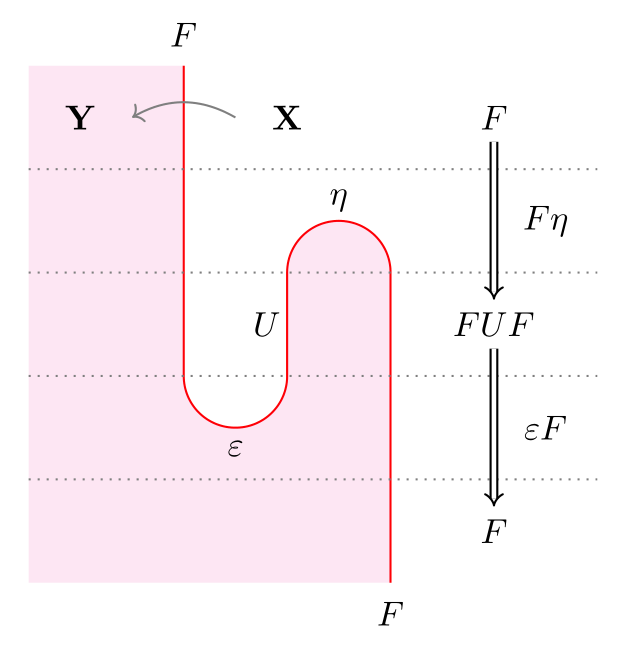Most diagrams in this post will not be as meticulously labelled as the above. Unlabelled white regions will always stand for a fixed category $\mathbf{X}$. If $F \dashv U$, I’ll use the same colored string to denote them both, since they can be distinguished from their context: above, $F$ goes from a white to red region, whereas $U$ goes from red to white (remember to read from right to left!). The composite monad $UF$ (not shown above) would also be a string of the same color, going from a white region to a white region.

Motivating examples

Example 1: Let $S$ be the free monoid monad and $T$ be the free abelian group monad over $\mathbf{Set}$. Then the elementary school fact that multiplication distributes over addition means we have a function $STX \to TSX$ for $X$ a set, sending $(a+b)(c+d)$, say, to $ac+ad+bc+bd$. Further, the composition of $T$ with $S$ is the free ring monad, $TS$.

Example 2: Let $A$ and $B$ be monoids in a braided monoidal category $(\mathcal{V}, \otimes, 1)$. Then $A \otimes B$ is also a monoid, with multiplication:

$A \otimes B \otimes A \otimes B \xrightarrow{A \otimes tw_{BA} \otimes B} A \otimes A \otimes B \otimes B \xrightarrow{\mu_A \otimes \mu_B} A \otimes B,$

where $tw_{BA}: B \otimes A \to A \otimes B$ is provided by the braiding in $\mathcal{V}$.

In example 1, there is also a monoidal category in the background: the category $\left(\mathbf{End}(\mathbf{Set}), \circ, \text{Id}\right)$ of endofunctors on $\mathbf{Set}$. But this category is not braided – which is why we need distributive laws!

Distributive laws, composite and lifted monads

Let $(S,\eta^S, \mu^S)$ and $(T,\eta^T,\mu^T)$ be monads on a category $\mathbf{X}$. I’ll use Scarlet and Teal strings to denote $S$ and $T$, resp., and white regions will stand for $\mathbf{X}$.

A distributive law of $S$ over $T$ is a natural transformation $\ell:ST \Rightarrow TS$, denoted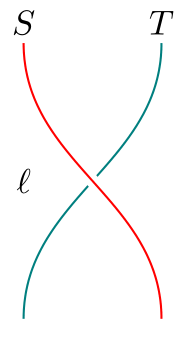satisfying the following equalities: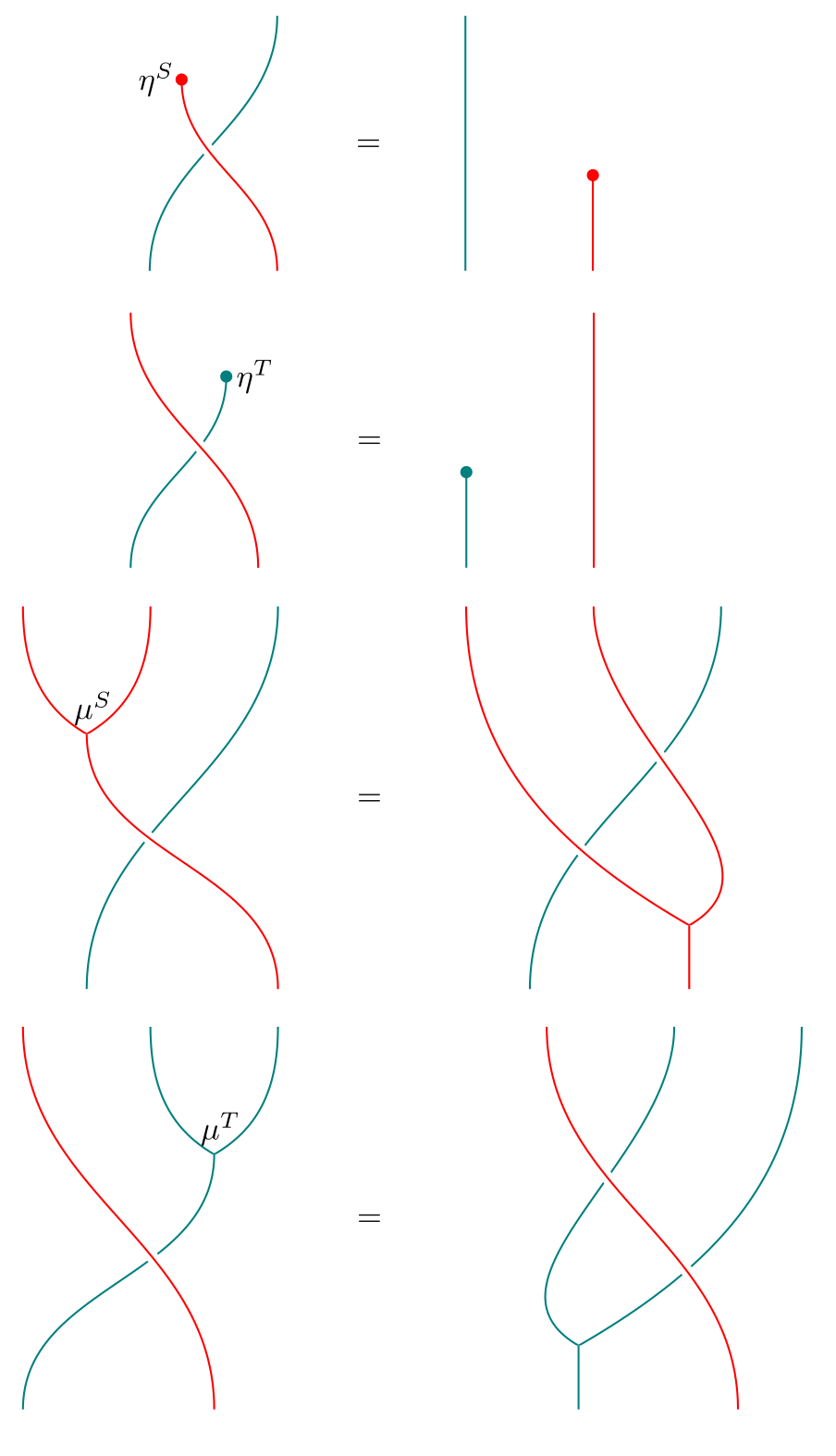A distributive law looks somewhat like a braiding in a braided monoidal category. In fact, it is a local pre-braiding: “local” in the sense of being defined only for $S$ over $T$, and “pre” because it is not necessarily invertible.

As the above examples suggest, a distributive law allows us to define a multiplication $m:TSTS \Rightarrow TS$: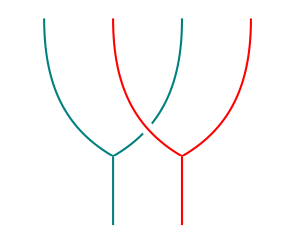It is easy to check visually that this makes $TS$ a monad, with unit $\eta^T \eta^S$. For instance, the proof that $m$ is associative looks like this: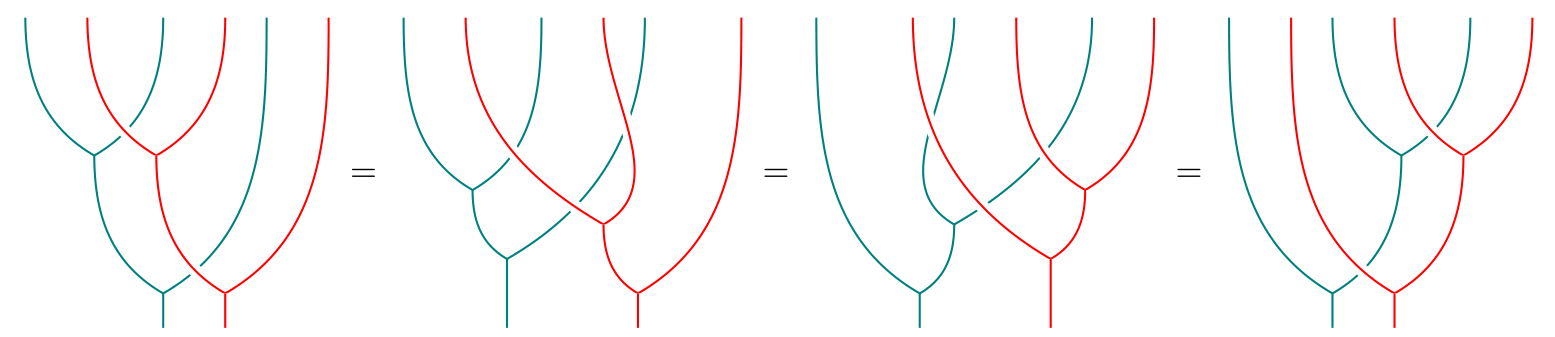Not only is $TS$ a monad, we also have monad maps $T \eta^S: T \Rightarrow TS$ and $\eta^T S: S \Rightarrow TS$: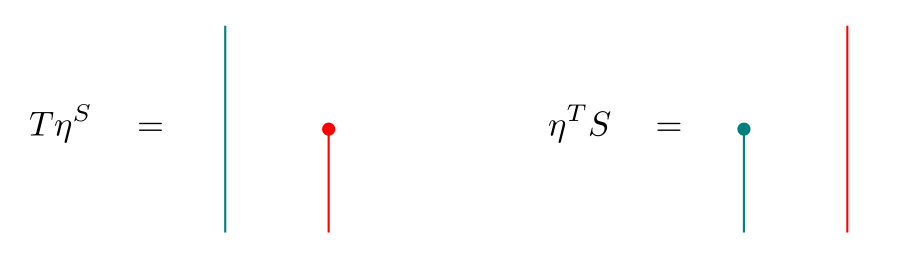Asserting that $T \eta^S$ is a monad morphism is the same as asserting these two equalities:Similar diagrams hold for $\eta^T S$. Finally, the multiplication $m$ also satisfies a middle unitary law: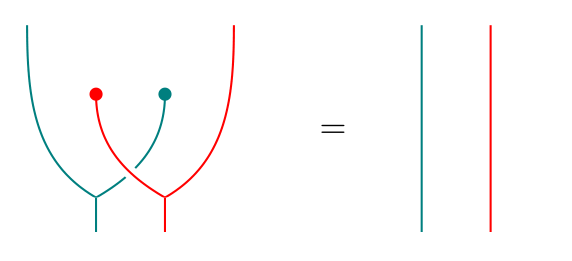To get back the distributive law, we can simply plug the appropriate units at both ends of $m$: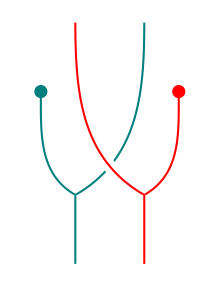This last procedure (plugging units at the ends) can be applied to any $m':TSTS \Rightarrow TS$. It turns out that if $m'$ happens to satisfy all the previous properties as well, then we also get a distributive law. Further, the (distributive law $\to$ multiplication) and (multiplication $\to$ distributive law) constructions are mutually inverse:

Theorem   The following are equivalent: (1) Distributive laws $\ell:ST \Rightarrow TS$; (2) multiplications $m:TSTS \Rightarrow TS$ such that $(TS, \eta^T \eta^S, m)$ is a monad, $T\eta^S$ and $\eta^T S$ are monad maps, and the middle unitary law holds.

In addition to making $TS$ a monad, distributive laws also let us lift $T$ to the category of $S$-algebras, $\mathbf{X}^S$. Before defining what we mean by “lift”, let’s recall the universal property of $\mathbf{X}^S$: Let $\mathbf{Y}$ be another category; then there is an isomorphism of categories between $\mathbf{Funct}(\mathbf{Y}, \mathbf{X}^S)$ – the category of functors $\tilde{G}: \mathbf{Y} \to \mathbf{X}^S$ and natural transformations between them, and $S$-$\mathbf{Alg}(\mathbf{Y})$ – the category of functors $G: \mathbf{Y} \to \mathbf{X}$ equipped with an $S$-action $\sigma: SG \Rightarrow G$ and natural transformations that commute with the $S$-action.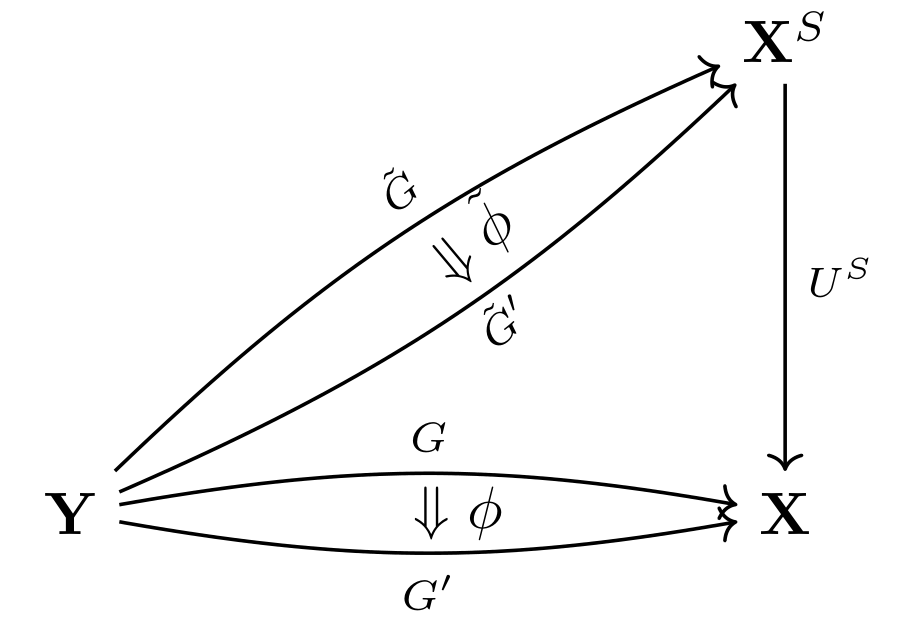Given $\tilde{G}: \mathbf{Y} \to \mathbf{X}^S$, we get a functor $\mathbf{Y} \to \mathbf{X}$ by composing with $U^S$. This composite $U^S \tilde{G}$ has an $S$-action given by the canonical action on $U^S$. The universal property says that every such functor $G: \mathbf{Y} \to \mathbf{X}$ with an $S$-action is of the form $U^S \tilde{G}$. Similar statements hold for natural transformations. We will call $\tilde{G}$ and $\tilde{\phi}$ lifts of $G$ and $\phi$, resp.

A monad lift of $T$ to $\mathbf{X}^S$ is a monad $(\tilde{T}, \tilde{\eta}^T,\tilde{\mu}^T)$ on $\mathbf{X}^S$ such that

$U^S \tilde{T} = T U^S, \qquad U^S \tilde{\eta}^T = \eta^T U^S, \qquad U^S \tilde{\mu}^T = \mu^T U^S.$

We may express $U^S \tilde{T} = T U^S$ via the following equivalent commutative diagrams: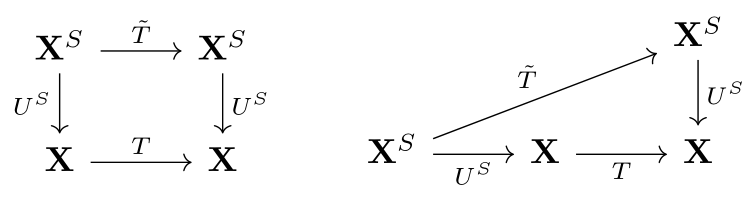The diagram on the right makes it clear that $\tilde{T}$ being a monad lift of $T$ is equivalent to $\tilde{T}, \tilde{\eta}^T, \tilde{\mu}^T$ being lifts of $TU^S, \eta^T U^S,\mu^T U^S$, resp. Thus, to get a monad lift of $T$, it suffices to produce an $S$-action on $TU^S$ and check that it is compatible with $\eta^T U^S$ and $\mu^T U^S$. We may simply combine the distributive law with the canonical $S$-action on $U^S$ to obtain the desired action on $TU^S$: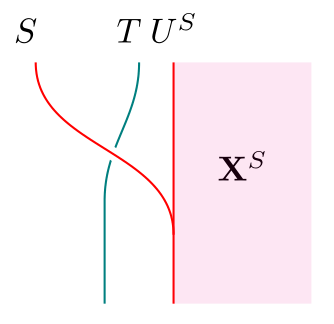(Recall that the unlabelled white region is $\mathbf{X}$. In subsequent diagrams, we will leave the red region unlabelled as well, and this will always be $\mathbf{X}^S$. Similarly, teal regions will denote $\mathbf{X}^T$.)

Conversely, suppose we have a monad lift $\tilde{T}$ of $T$. Then the equality $U^S \tilde{T} = T U^S$ can be expressed by saying that we have an invertible natural transformation $\chi: U^S \tilde{T} \Rightarrow TU^S$. Using $\chi$ and the unit and counit of the adjunction $F^S \dashv U^S$ that gives rise to $S$, we obtain a distributive law of $S$ over $T$: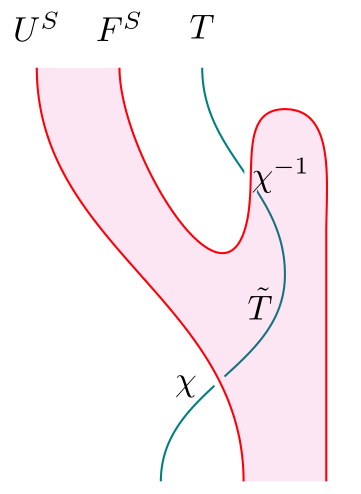The key steps in the proof that these constructions are mutually inverse are contained in the following two equalities: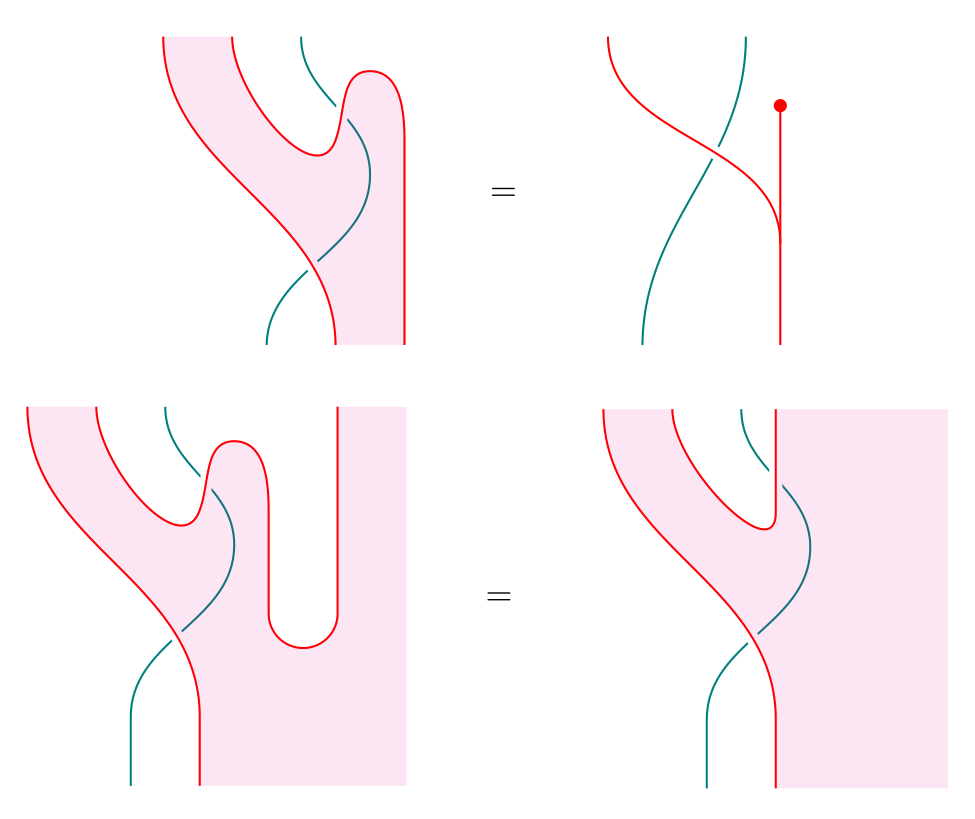The first shows that the resulting distributive law in the (distributive law $\to$ monad lift $\to$ distributive law) construction is the same as the original distributive law we started with. The second shows that in the (monad lift $\tilde{T}$ $\to$ distributive law $\to$ another lift $\tilde{T}'$) construction, the $S$-action on $U^S \tilde{T}'$ (LHS of the equation) is the same as the original $S$-action on $U^S \tilde{T}$ (RHS), hence $\tilde{T} = \tilde{T}'$ (by virtue of being lifts, $\tilde{T}$ and $\tilde{T}'$ can only differ in their induced $S$-actions on $U^S \tilde{T} = U^S \tilde{T}' = TU^S$). We thus have another characterization of distributive laws:

Theorem   The following are equivalent: (1) Distributive laws $\ell:ST \Rightarrow TS$; (3) monad lifts of $T$ to $\mathbf{X}^S$.

In fact, the converse construction did not rely on the universal property of $\mathbf{X}^S$, and hence applies to any adjunction giving rise to $S$ (with a suitable definition of a monad lift of $T$ in this situation). In particular, it applies to the Kleisli adjunction $F_S \dashv U_S$. Since the Kleisli category $\mathbf{X}_S$ is equivalent to the subcategory of free $S$-algebras (in the classical sense) in $\mathbf{X}^S$, this means that to get a distributive law of $S$ over $T$, it suffices to lift $T$ to a monad over just the free $S$-algebras! (Thanks to Jonathan Beardsley for pointing this out!) The resulting distributive law may be used to get another lift of $T$, but we should not expect this to be the same as the original lift unless the original lift was “monadic” to begin with, in the sense of being a lift to $\mathbf{X}^S$.

There are two further characterizations of distributive laws that are not mentioned in Beck’s paper, but whose equivalences follow easily. Eugenia Cheng in Distrbutive laws for Lawvere theories states that distributive laws of $S$ over $T$ are also equivalent to extensions of $S$ to a monad $\tilde{S}$ on the Kleisli category $\mathbf{X}_T$. This follows by duality from the above theorem, since $\mathbf{X}_T = (\mathbf{X}^{op})^T$. Finally, Ross Street’s The formal theory of monads (which was also covered in a previous Kan Extension Seminar post) says that distributive laws in a $2$-category $\mathbf{K}$ are precisely monads in $\mathbf{Mnd}(\mathbf{K})$. It is a fun and easy exercise to draw string diagrams for objects of $\mathbf{Mnd}(\mathbf{Mnd}(\mathbf{K}))$; it becomes visually obvious that these are the same as distributive laws.

After characterizing distributive laws, Beck characterizes the algebras for the composite monad $TS$.

Just as a morphism of rings $R \to R'$ induces a “restriction of scalars” functor $R'$-$\mathbf{Mod} \to R$-$\mathbf{Mod}$, the monad maps $T \eta^S: T \Rightarrow TS$ and $\eta^T S: S \Rightarrow TS$ induce functors $\hat{U}^{TS}: \mathbf{X}^{TS} \to \mathbf{X}^T$ and $\tilde{U}^{TS}: \mathbf{X}^{TS} \to \mathbf{X}^S$.

Equivalently, we have $S$- and $T$-actions on $U^{TS}$, which we call $\sigma: SU^{TS} \Rightarrow U^{TS}$ and $\tau: T U^{TS} \Rightarrow U^{TS}$. Let $\varepsilon: TS\, U^{TS} \Rightarrow U^{TS}$ be the canonical $TS$-action on $U^{TS}$. The middle unitary law then implies that $\varepsilon = T\sigma \cdot \tau$: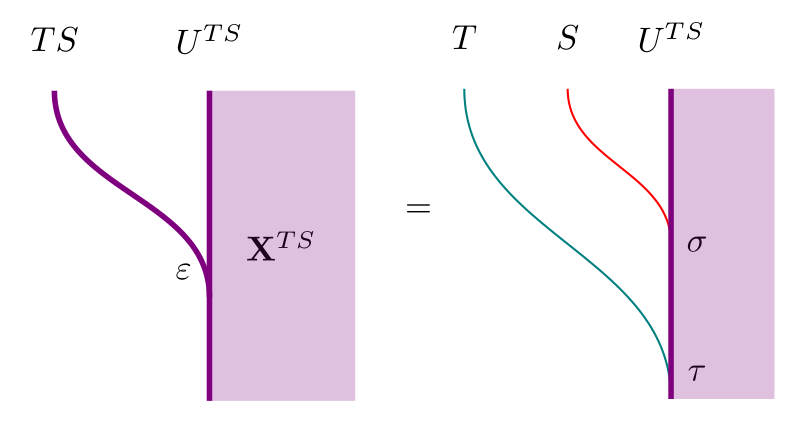Further, $\sigma$ is distributes over $\tau$ in the following sense: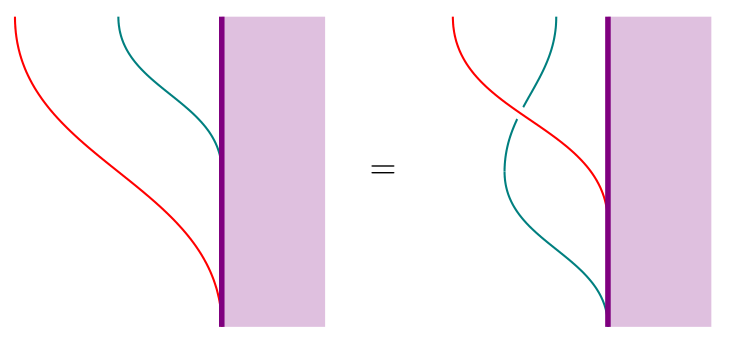The properties of these actions allow us to characterize $TS$-algebras:

Theorem   The category of algebras for $TS$ coincides with that of $\tilde{T}$:

$\mathbf{X}^{TS} \cong (\mathbf{X}^S)^{\tilde{T}}$

To prove this, Beck constructs $\Phi: (\mathbf{X}^{S})^{\tilde{T}} \to \mathbf{X}^{TS}$ and its inverse $\Phi^{-1}: \mathbf{X}^{TS} \to (\mathbf{X}^S)^{\tilde{T}}$. These constructions are best summarized in the following diagram of lifts: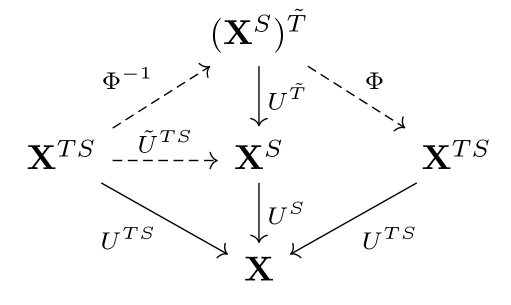On the left half of the diagram, we see that to get $\Phi^{-1}$, we must first produce a functor $\tilde{U}^{TS}: \mathbf{X}^{TS} \to \mathbf{X}^S$ with a $\tilde{T}$-action. We already have $\tilde{U}^{TS}$ as a lift of $U^{TS}$, given by the $S$-action $\sigma$. We also have the $T$-action $\tau$ on $U^{TS}$, which $\sigma$ distributes over. This is precisely what is required to get a lift of $\tau$ to a $\tilde{T}$-action $\tilde{\tau}$ on $\tilde{U}^{TS}$, which gives us $\Phi^{-1}$.

On the right half of the diagram, to get $\Phi$ we need to produce a functor $(\mathbf{X}^{S})^{\tilde{T}} \to \mathbf{X}$ with a $TS$-action. The obvious functor is $U^S U^{\tilde{T}}$, and we get an action by using the canonical actions of $S$ on $U^S$ and $\tilde{T}$ on $U^{\tilde{T}}$: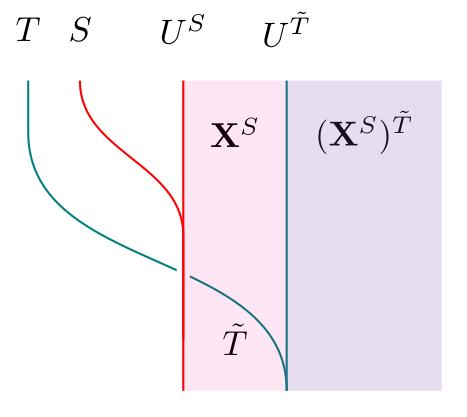All that’s left to prove the theorem is to check that $\Phi$ and $\Phi^{-1}$ are inverses. In a similar fashion, we can prove the dual statement (again found in Cheng’s paper but not Beck’s):

Theorem   The Kleisli category of $TS$ coincides with that of $\tilde{S}$:

$\mathbf{X}_{TS} \cong (\mathbf{X}_T)_{\tilde{S}}$

From now on, we identify $\mathbf{X}^{TS}$ with $(\mathbf{X}^S)^{\tilde{T}}$. Under this identification, it turns out that $\tilde{U}^{TS} \cong U^{\tilde{T}}$, and we obtain what Beck calls a distributive adjoint situation comprising 3 pairs of adjunctions: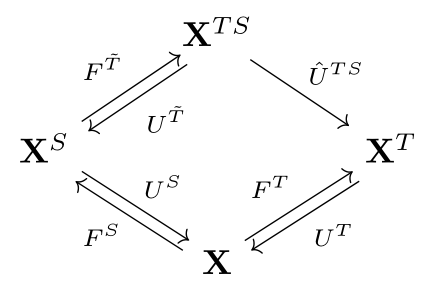For this to qualify as a distributive adjoint situation, we also require that both composites from $\mathbf{X}^{TS}$ to $\mathbf{X}$ are naturally isomorphic, and both composites from $\mathbf{X}^S$ to $\mathbf{X}^T$ are naturally isomorphic. This can be expressed in the following diagram by requiring both blue circles to be mutually inverse, and both red circles to be mutually inverse: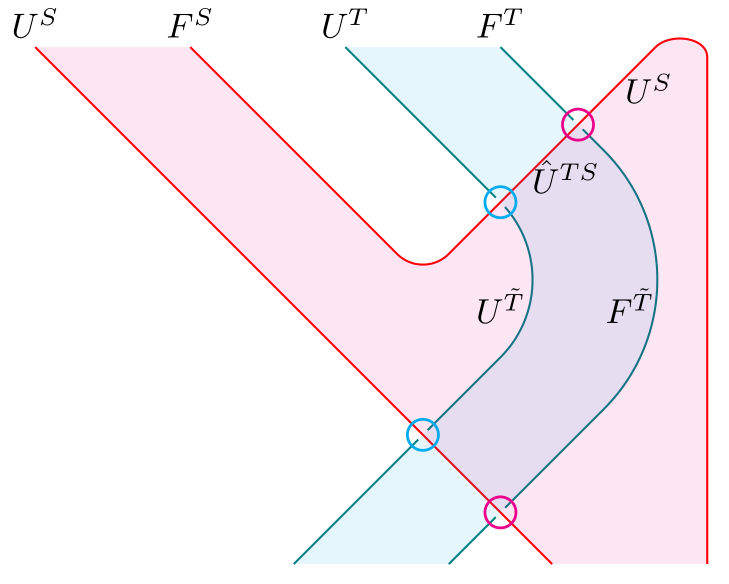(Recall that colored regions are categories of algebras for the corresponding monads, and the cap and cup are the unit and counit of $F^S \dashv U^S$.)

This diagram is very similar to the diagram for getting a distributive law out of a lift $\tilde{T}$, and it is easy to believe that any such distributive adjoint situation (with 3 pairs of adjoints - not necessarily monadic - and the corresponding natural isomorphisms) leads to a distributive law.

Finally, suppose the “restriction of scalars” functor $\hat{U}^{TS}$ has an adjoint. This adjoint behaves like an “extension of scalars” functor, and Beck fittingly calls it $(\,) \otimes_S F^T$ at the start of his paper. I’ll use $\hat{F}^{TS}$ instead, to highlight its relationship with $\hat{U}^{TS}$.

In such a situation, we get an adjoint square consisting of 4 pairs of adjunctions. By drawing these 4 adjoints in the following manner, it becomes clear which natural transformations we require in order to get a distributive law: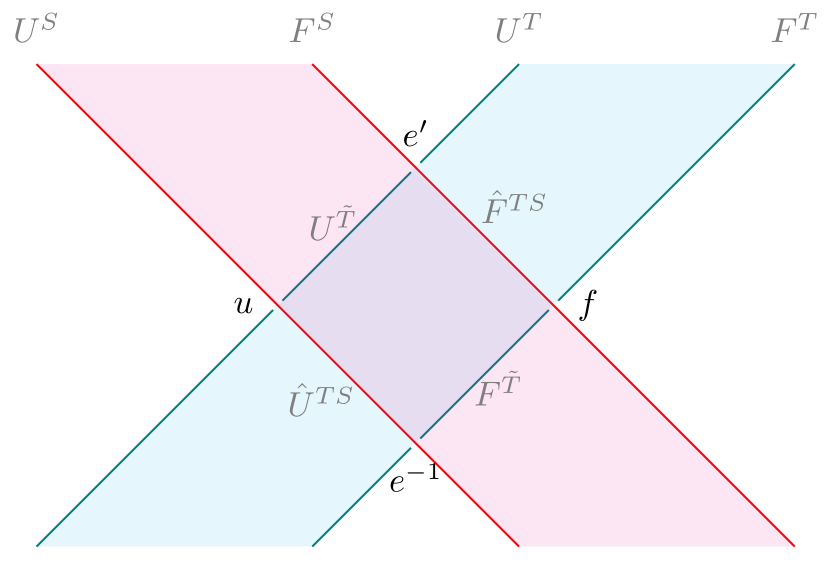(Recall that $S = U^S F^S$ and $T = U^T F^T$, so this is a “thickened” version of what a distributive law looks like.)

It turns out that given the natural transformation $u$ between the composite right adjoints $U^S U^{\tilde{T}}$ and $U^T \hat{U}^{TS}$, we can get the natural transformation $f$ as the mate of $u$ between the corresponding composite left adjoints $\hat{F}^{TS} F^T$ and $F^{\tilde{T}} F^S$. Note that $f$ is invertible if and only if $u$ is. We may use $u$ or $f$, along with the units and counits of the relevant adjunctions, to construct $e$: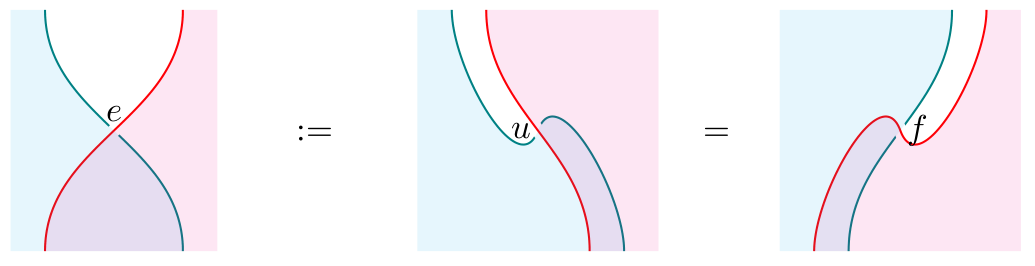But $e$ is in the wrong direction, so we have to further require that $e$ is invertible, to get $e^{-1}$. We get $e'$ from $u^{-1}$ or $f^{-1}$ in a similar manner. Since $e'$ will turn out to already be in the right direction, we will not require it to be invertible. Finally, given any 4 pairs of adjoints that look like the above, along with natural transformations $u,f,e,e'$ satisfying the above properties, we will get a distributive law!

What next?

Beck ends his paper with some examples, two of which I’ve already mentioned at the start of this post. During our discussion, there were some remarks on these and other examples, which I hope will be posted in the comments below. Instead of repeating those examples, I’d like to end by pointing to some related works:

• Since we’ve been talking about Lawvere theories, we can ask what distributive laws look like for Lawvere theories. Cheng’s Distributive laws for Lawvere theories, which I’ve already referred to a few times, does exactly that. But first, she comes up with 4 settings in which to define Lawvere theories! She also has a very readable introduction to the correspondence between Lawvere theories and finitary monads.

• As Beck mentions in his paper, we can similarly define distributive laws between comonads, as well as mixed distributive laws between a monad and a comonad. Just as we can define bimonoids/bialgebras, and thus Hopf monoids/algebras, in a braided monoidal category, such distributive laws allow us to define bimonads and Hopf monads. There are in fact two distinct notions of Hopf monads: the first is described in this paper by Alain Bruguières and Alexis Virelizier (with a follow-up paper coauthored with Steve Lack, and a diagrammatic approach with amazing surface diagrams by Simon Willerton); the second is this paper by Bachuki Mesablishvili and Robert Wisbauer. The difference between these two approaches is described in the Mesablishvili-Wisbauer paper, but both involve mixed distributive laws. Gabriella Böhm also recently gave a talk entitled The Unifying Notion of Hopf Monad, in which she shows how the many generalizations of Hopf algebras are just instances of Hopf monads (in the first sense) in an appropriate monoidal bicategory!

• We also saw that distributive laws are monads in a category of monads. Instead of thinking of distributive laws as merely a means of composing monads, we can study distributive laws as objects in their own right, just as monoids in a category of monoids (a.k.a. abelian monoids) are studied in their own right! The story for monoids terminates at this step: monoids in abelian monoids are just abelian monoids. But for distributive laws, we can keep going! See Cheng’s paper on Iterated Distributive Laws, where she shows the connection between iterated distributive laws and $n$-categories. In addition to requiring distributive laws between each pair of monads involved, it is also necessary to have a Yang-Baxter equation between every three monads: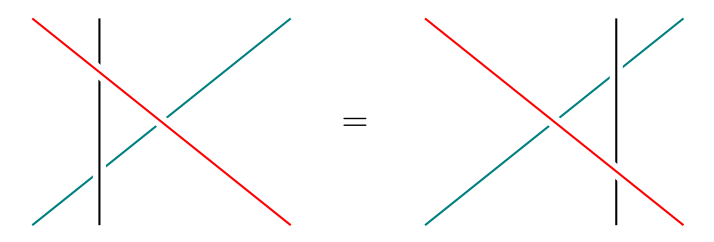• Finally, there seems to be a strange connection between distributive laws and factorization systems (e.g. here, here and even in “Distributive Laws for Lawvere theories” mentioned above). I can’t say more because I don’t know much about factorization systems, but hopefully someone else can say something illuminating about this!
Posted at February 18, 2017 7:06 AM UTC

TrackBack URL for this Entry:   https://golem.ph.utexas.edu/cgi-bin/MT-3.0/dxy-tb.fcgi/2940

Re: Distributive Laws

Thanks Ze for a great post! I felt like I’ve gained something from seeing the commutative diagrams presented as string diagrams, in particular the intuition of distributive laws as local non-invertible braidings.

This makes me wonder if in the past I’ve paid too much heed to shapes of diagrams. For instance, if I were to describe the distributive law axioms to another category theorist but couldn’t be bothered to spell them out, I’d say that a distributive law between two monads $T$ and $S$ on the same object is given by a 2-cell $\ell \colon ST \Rightarrow TS$ satisfying two triangles involving the units of the monads and two pentagons involving the multiplications of the monads.

My question: do the shapes of these commutative diagrams (the fact that two involve three morphisms and the other two involve five) have any significance?

Posted by: Emily Riehl on February 20, 2017 11:25 PM | Permalink | Reply to this

Re: Distributive Laws

In this case, I would tend to say that all four of the diagrams are actually rectangles; it’s just that one of their sides might consist of two or zero morphisms. From an unbiased perspective, a distributive law should satisfy $2\omega$ axioms saying that it commutes with all the operations of a monad (= a monoid in an endofunctor category), of which there is exactly one $n$-ary one for each $n$, and each such axiom is naturally regarded as a commutativity rectangle (down-then-right = right-then-down) even if some of the sides have more than one morphism in them. It just so happens that, as usual, the cases $n=0,2$ suffice.

Posted by: Mike Shulman on February 21, 2017 12:14 PM | Permalink | Reply to this

Re: Distributive LawsThere is a comment at the end of Beck’s paper that I’d like to understand better.

A monad on Set is called inconsistent if it is either of the two monads corresponding to $h$-levels $-2$ or $-1$ in homotopy type theory, by which I mean:

• it is the terminal monad $T(X) := 1$ for all $X$, or

• it is the monad with $T(0) := 0$ and $T(X) := 1$ otherwise.

Beck continues:

It is known that if $T$ is a consistent monad in sets, then the unit $\eta_X \colon X \to TX$ is a monomorphism for every $X$. And every monad in sets, as a functor, preservers monomorphisms.

I don’t see how to prove either of these statements. (Note that if $X$ admits a $T$-algebra structure, then $\eta_X$ must be a split monomorphism, but I can’t see how to get from this to the general argument.)

The reason these observations are interesting is that it follows in particular that the monad maps $\eta_S \colon S \Rightarrow TS$ and $T\eta \colon T \Rightarrow TS$ are component wise monomorphisms, which means that

the operations of $S$ and of $T$ are mapped injectively into operations of $ST$, and no new equations hold among them in the composite.

Posted by: Emily Riehl on February 20, 2017 11:40 PM | Permalink | Reply to this

Re: Distributive Laws

For the first statement, if $T$ has at least one algebra with at least two elements, then the unit map $\eta_X : X \to T X$ is injective for each set $X$. For take distinct $x, y \in X$, and choose an algebra $A$ with at least two elements $a, b$. We can then pick a function $f: X \to A$ with $f(x) = a$ and $f(y) = b$. By adjointness, $f$ factors as $\eta_X$ followed by some map $T X \to A$. Since $f(x) \neq f(y)$, it follows that $\eta_X(x) \neq \eta_X(y)$.

So for the first statement, all that remains to prove is that if every $T$-algebra has $\leq 1$ element then $T$ is one of Beck’s two “inconsistent” monads. To see this, take a set $X$. The set $T(X)$ can be given the structure of a $T$-algebra, so it has either no elements or one element. But if $X$ is nonempty then $T(X)$ must be nonempty too, since we have the unit map $X \to T(X)$. So $T(X) = 1$ for all nonempty $X$. This leaves only the two options listed.

The second statement is that the underlying endofunctor $T$ of every monad on $Set$ preserves monomorphisms. Well, every mono in $Set$ is split unless its domain is empty, so we only need to show that $T(0 \to X)$ is mono for all sets $X$. And it’s enough to show that $T(0 \to 1)$ is mono, since it factors through $T(0 \to X)$. But now I’m stuck.

Posted by: Tom Leinster on February 21, 2017 12:13 AM | Permalink | Reply to this

Re: Distributive Laws

OK, here’s a proof that for every monad $(T, \eta, \mu)$ on $Set$, the endofunctor $T$ preserves monics. It’s not the most elegant proof in the world — maybe someone can improve on it.

• In $Set$, every monic with nonempty domain is split. So it suffices to show that $T(0 \to X)$ is monic for all sets $X$.

• If $T(0)$ is empty, this is immediate. So assume that $T(0)$ is nonempty.

• Let $X$ be any set. By the assumption just made, we can pick a map $X \to T(0)$. Then the unique map $0 \to T(0)$ factorizes as $0 \to X \to T(0)$. Applying $T$ to this factorization gives $\Bigl( T(0) \stackrel{T(!)}{\to} T^2(0) \Bigr) = \Bigl( T(0) \stackrel{T(!)}{\to} T(X) \to T^2(0) \Bigr).$ If the composite of two maps is monic then so is the first of those maps. So it is enough to prove that $T(!): T(0) \to T^2(0)$ is monic.

• The map $!$ just mentioned is the unique map $0 \to T(0)$. But this is $\eta_0$. So it is enough to ptove that $T(\eta_0)$ is monic. And this is true, since by definition of monad, $T(\eta_0)$ is split by $\mu_0$.

Posted by: Tom Leinster on February 21, 2017 1:10 AM | Permalink | Reply to this

Re: Distributive Laws

In this last case where $T 0$ is nonempty you can directly construct a splitting for $T!_X \colon T 0 \to T X$, as follows. Let $f \colon X \to T 0$ be your map such that $\eta_0 = f \circ !_X$. Then since $\mu_0 \circ T f \circ T!_X = \mu_0 \circ T \eta_0 = 1_{T 0}$ we see that the composite $\mu_0 \circ T f$ splits $T!_X$, which is therefore monic.

Posted by: Alexander Campbell on February 21, 2017 1:48 AM | Permalink | Reply to this

Re: Distributive Laws

Here’s a challenge: can anyone give a constructive proof of (a version of) either of these statements, or an argument that they fail constructively?

Constructively, there are other monads on Set that one probably ought to exclude along with the “inconsistent” ones (although I don’t see what is “inconsistent” about the propositional truncation). For instance, any Lawvere-Tierney topology on the set of truth values gives rise to a subtopos of Set, which is in particular a reflective subcategory and thus an idempotent monad, and its units will not always be monic (but it will preserve monos, since it is left exact). Classically, the only proper subtopos of Set is the trivial one, but constructively there can be others. However, these clearly aren’t the sort of monads the statements are meant to apply to; is there a natural way to exclude them?

Posted by: Mike Shulman on February 21, 2017 11:43 AM | Permalink | Reply to this

Re: Distributive Laws

I know, right? I learned how to do this kind of argument from Peter Johnstone’s Cambridge Part III Category Theory problem sheets, which I tutored for a year or two. It took me a while to get the hang of them.

Eventually, I realized the huge gap between the style of argument you use for a general category and the style of argument you use for a particular category (such as the category of sets). When you’re told that you’re working with a particular category, it’s no holds barred: you use whatever you can. Learning to really take advantage of that assumption was difficult for me (and doubtless I haven’t mastered it yet). It’s the opposite of doing everything in a scrupulously constructive manner.

Of course, it’s a bonus if you can show that the argument works for an arbitrary topos, or regular category, or adhesive quasi-topos, or whatever — and Peter himself is very good at that kind of thing. But that comes second.

Posted by: Tom Leinster on February 21, 2017 11:27 PM | Permalink | Reply to this

Re: Distributive Laws

While I agree that there’s a difference between arguing in a general category and a particular one, I wouldn’t identify it with constructivity versus classicality. A philosophical constructivist would dispute that your argument works even for the particular category $\mathbf{Set}$.

Posted by: Mike Shulman on February 22, 2017 3:27 AM | Permalink | Reply to this

Re: Distributive Laws

While I agree that there’s a difference between arguing in a general category and a particular one, I wouldn’t identify it with constructivity versus classicality.

Nor would I.

Posted by: Tom Leinster on February 22, 2017 10:05 PM | Permalink | Reply to this

Re: Distributive Laws

Those string diagrams look beautiful. Which software did you use?

Posted by: Anonymous Coward on February 21, 2017 9:11 AM | Permalink | Reply to this

Re: Distributive Laws

Ze can probably give you more information about it but in case he does not see your question, here is what he told us live: the diagram are done with TikZ, with the help of a software called TikzEdt.

Posted by: Pierre Cagne on February 21, 2017 11:42 AM | Permalink | Reply to this

Re: Distributive Laws

Thanks! As Pierre says, they were done in TikZ (I took screenshots of the resulting PDF). The use of TikzEdt made it a lot easier, because I could select multiple nodes and drag them around. This meant that I could pretty much treat the strings as real strings, and just drag one across another to form the necessary braidings.

To get the effect of a line crossing over another line, I used Tikz’s “double” property for lines.

Posted by: Ze on February 22, 2017 12:05 AM | Permalink | Reply to this

Re: Distributive Laws

Nice post, nice pictures! In the believe that beautiful theory is enhanced by a wide variety of examples, and bearing in mind (a) that people tend to mention the ring monad as the key example of a distributive law and (b) that I should look at the Hopf monad example, can I ask what other interesting examples there are?

Posted by: Simon Willerton on February 21, 2017 11:15 AM | Permalink | Reply to this

Re: Distributive Laws

In our discussion, David Myers pointed out that Zappa-Szep products of groups $H,K$ are precisely distributive laws between the monads $T = H \times -$ and $S = K \times -$. These include semi-direct and direct products as special cases.

The characterization of distributive laws as multiplications making $T S$ into a monad translates into the following: a group $G$ is a Zappa-Szep product of subgroups $H$ and $K$ iff $G = H K$ and $H \cap K = \{e_G\}$, where $e_G$ is the identity of $G$.

Saying that $G = H K$ is the same as saying we have group structure on the set $H \times K$. That $H$ and $K$ are subgroups of $G$ corresponds to the monad maps $T \eta^S$ and $\eta^T S$ being monomorphisms. The unit of $T S$ is $\eta^T \eta^S$, which makes $e_H \times e_K$ the unit of $G$. In this case, the diagram that defines the unit of $T S$ is a pullback diagram (of $\eta^T S$ and $T \eta^S$), and thus $H \cap K = \{e_G\}$.

I’m not sure if the diagram that defines the unit of $T S$ is always a pullback for more general $T$ and $S$, but if $T$ and $S$ are consistent, we at least have monomorphisms $\eta^T S$ and $T \eta^S$, so $T$ and $S$ can be thought of as ‘submonads’ of $TS$. To get direct and semi-direct products, we would need something like ‘normal submonads’, but I don’t know what that would look like.

We can also work with monads of the form $M \otimes -$ for monoids $M$ in other monoidal categories, and I think bicrossed products and bismash products of Hopf algebras are examples of this.

David also pointed out that expressing $G$ as $H K$ gives a ‘factorization’ of $G$. This is in fact related to strict factorization systems on categories (see section 4 of this). Small categories are monads in $\textbf{Span}$, and distributive laws between them yield ‘composite’ categories with strict factorization systems given by the original categories. If we think of a group as a 1 object category containing only isomorphisms, then a strict factorization system on that category gives the decomposition that we saw above.

Distributive laws between a monad and comonad also turn up in algebraic weak factorization systems, but I’m not sure if they arise from distributive laws in the same manner that strict factorization systems do.

Posted by: Ze on February 22, 2017 2:10 AM | Permalink | Reply to this

Re: Distributive Laws

Thanks Ze for writing this up! I’ll just mention a few other examples and special cases.

I never learned about Zappa-Szep products in my algebra classes, but I did learn about two special cases: the semi-direct product, and the direct product. An internal Zappa-Szep product happens when the group $G$ factors as the product of two disjoint subgroups $HK$. This product is semi-direct when $H$ is normal, and is direct when both $H$ and $K$ are normal. This tells us, for example, that if an abelian group factors as a semi-direct product of two other abelian groups (or even as a Zappa-Szep product), then it is actually their direct product.

It’s pretty straightforward to see how the direct product gives a distributive law between two groups $H$ and $K$. We define the law $H \times K \to K \times H$ to be the twist map $(h, k) \mapsto (k, h)$, and the multiplication induced is the composite $(h, k, h', k') \mapsto (h, h', k, k') \mapsto (hh', kk')$ which is exactly the multiplication you want for the direct product.

I found the semi-direct product case revealing as well. When I was learning about semi-direct products, I wondered why we only acted on one of the factors. Seeing it as a distributive law makes it clear: we only need to twist the middle two instances of the groups like this $H \times K \times H \times K \to H \times H \times K \times K$, so we only need to act on that copy of $H$ that moved. So, explicitly, let $\phi : K \to \text{Aut}(H)$ be a homomorphism. Define the distributive law by $(h, k) \mapsto (k, \phi(k)(h))$. The multiplication on the composite group then becomes $(h, k, h', k') \mapsto (h, \phi(k)(h'), k, k') \mapsto (h \cdot \phi(k)(h'), kk')$ which is the definition of the multiplication in the semi-direct product.

More fun than distributive laws between groups are distributive laws between algebraic structures of different kinds. The functor sending a group $G$ to the monad $G \times -$ of its actions is fully faithful, as is the functor sending a ring to the monad of its modules. We can recover the group $G$ from $G \times -$ with its monad structure as $G \times 1$, and the ring as the free module on $1$ generator. So we are justified in equating a group with its monad of actions, and a ring with its monad of modules. If $G$ is a group and $R$ a ring, then we can distribute $G$ over $R$ by the law $GRX \to RGX$ sending $(g, \sum r_i x_i) \mapsto \sum r_i (g, x_i)$. This gives us another ring, the group ring $R[G]$, which we can see by checking the action on $1$: the elements of $RG1$ are formal $R$-linear combinations $\sum r_i g_i$ of group elements. The modules of $R[G]$ are representations of $G$ on $R$-modules.

On a related note, I’d be really interested in seeing a characterization of all the categories of algebras (by which I mean categories of modules for a monad) which embed into the category of monads. I’m particularly interested when the base is the category of sets, but it would be great to know over a general category as well.

One last example, less algebraic this time. Let $P$ be the powerset monad (whose unit is the inclusion of singletons, and whose multiplication is union), and $M$ the monoid monad. We can define a distributive law $MP \to PM$ by sending a formal product $H_1 \cdots H_n$ of subsets to the set $\{h_1 \cdots h_n \mid h_i \in H_i\}$ of formal products of their elements. This is the usual set product, the one we were using to talk about Zappa-Szep products. Since the modules of the powerset monad are sup-lattices, I’d expect the modules of $PM$ to be quantales, but I haven’t checked this.

Posted by: David Jaz Myers on February 24, 2017 9:52 PM | Permalink | Reply to this

Re: Distributive Laws

Just to add to this informative post and discussion. As part of a “pearl” paper we were writing, Maciej Pirog and I needed a concrete definition of when an algebraic theory is a composite of two algebraic theories.

If you’re interested, you can find our concrete definition on page 9 of this preprint (Definition 3).

Of course, our definition is inspired by Eugenia Cheng’s paper mentioned above. But I was surprised to find that the zig-zag condition in Eugenia’s work can be simplified in this concrete setting. To be honest, I’m also surprised that I can’t find our definition in print anywhere.

Part of the reason I’m writing this comment now is that we’re grateful for any feedback on this (although the paper is now accepted and we ought to submit a camera-ready version in the next week or so).

Posted by: Sam Staton on May 22, 2017 11:18 AM | Permalink | Reply to this

Re: Distributive Laws

I think the term pre-braiding in the text should be replaced by lax braiding.

Posted by: Martin Brandenburg on February 25, 2020 10:28 PM | Permalink | Reply to this

Post a New Comment